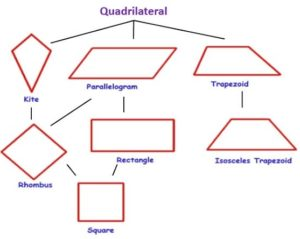# Polygons – 2D AND 3D Shapes

Last Updated on July 26, 2020 by Alabi M. S.

MATHEMATICS

Basic 6

TOPIC: Shapes

PERFORMANCE OBJECTIVES

By the end of the lesson, the pupils should have attained the following objectives (cognitive, affective and psychomotor) and should be able to –

1. name two and three dimensional shapes;

2. draw line of symmetry;

3. identify and appreciate 3 – dimensional shapes;

4. solve quantitative aptitude problems relating to polygon.

ENTRY BEHAVIOR

INSTRUCTIONAL MATERIALS

The teacher will teach the lesson with the aid of:

charts of two and three dimensional figures.

CONTENT OF THE LESSON

2 – DIMENSIONAL SHAPES NOT EXCEEDING OCTAGON

2 – Dimensional shapes have length and width (breadth). Polygons are 2-dimensional shapes.

Polygons have a straight sides and the shape is closed (all the lines connect up).

The name of each shape tells you the number of sides it has.

TYPES OF POLYGONS

A regular polygon has all angles equal and all sides equal, otherwise it is irregular.

TRIANGLE AND QUADRILATERAL

Triangle – Triangle is a three sided figure (shape). It is classified by number of sides and size of angles or both.Quadrilateral is four – sided figure (shape). There are special types of quadrilateral.LESSON EVALUATION

1. ___________ is a three sided figure.

a. Circle

b. Pentagon

c. Rectangle

d. Triangle

2. ___________ is a four sided figure.

a. Circle

b. Pentagon

c. Rectangle

d. Triangle

3. ___________ has an equal sides and angles.

a. Square

b. Rectangle

c. Kite

d. triangle

Draw the lines of symmetry on triangles and rectangles.

Mention the number of lines of symmetry of triangles and rectangles.
Pentagon

Pentagon means 5 sides, hexagon means 6, heptagon means 7 and octagon means 8.

The shape below is pentagon.

It has five sides.

LESSON EVALUATION

1. Draw the lines of symmetry each of the above figures.

2. Mention the number of lines of symmetry of each figures above.

3. Complete the table below:

3 – DIMENSIONAL SHAPES

3-Dimensional shapes have height, width and depth.

Examples are:

cube

cuboid

cylinder

sphere

cone

triangular prism

square – base pyramid

regular tetrahedron

Further exercise will be taken from the listed reference materials.

PRESENTATION

To deliver the lesson, the teacher adopts the following steps:

1. To introduce the lesson, the teacher revises the previous lesson- time. Based on this, he/she asks the pupils some questions;

2. Guides the pupils to recall the names and features of given shapes;

Pupils Activities – Identify a given plane shapes in terms of its name and essential features e.g. square, rectangle, triangle, rhombus, parallelograms etc.

3. Guides pupils to identify lines of symmetry, number of sides, number of angles, etc.

4. Pupils Activities – Using paper cut outs to determine the number of lines of symmetry, number of angles and number of sides.

5. Guides pupils relate 3-Dimensional to the world as around them;

Pupils Activities – Identify and appreciate 3-dimensional shapes e.g. Cube, cone, triangular, prison i.e. Closed or solid object. Examples of cube – sugar, maggi, cone, ice cream, cuboid – boxes, etc.

CONCLUSION

To conclude the lesson for the week, the teacher revises the entire lesson and links it to the following week’s lesson.

LESSON EVALUATION

Pupils to complete chart to indicate:

1. number of lines of symmetry;

2. number of sides;

3. Number of angles contained in a given shapes.

Smart Teachers Plan Lesson Notes - ClassRoomNotes support teachers with hands-on lesson plans/notes, printable and thoughtful teaching resources. @ClassRoomNotes - We always love to hear from you always. Stay connected with your classroom.
error: